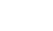# Most Tested New SAT Math Concepts

If the SAT math section looks all Greek to you, don’t worry – it’s not. It can be quite confusing to know where to start studying, especially since the SAT test makers decided to give weird names to each topic section such as ‘Passport to Advanced Math‘ and ‘Additional Topics.’

Fortunately, Magoosh is here to give you the inside scoop on how to decode what that all means. With 61% of the New SAT math topics being algebra-based, you should spend a good chunk of your time studying variables, equations, expressions, functions, and things of that nature.

## New SAT Math Concept 1: Linear Equations

Linear equations are exactly what they sound like: equations that represent straight lines. Because it is considered a basic, foundational math skill, you’ll be expected to know this stuff inside and out.

Here is an example of a linear equation problem you will see on the test:

If 6x = 42 and xk = 2, what is the value of k?

A. 2/7
B. 1/6
C. 7
D. 1/7
E. 5

If you feel shaky on this topic, I recommend getting a graphing calculator and fiddling around with the graphing tool. Try plugging in an equation and see what happens when you change a number here or there.

How does 3x = y compare to 2x = y? What about 2x = y – 2?

Next, go ahead and draw out linear equations on a sheet of graph paper. What does it mean when the equations cross each other? Can two equations cross each other more than once?

## New SAT Math Concept 2: Non-Linear Equations

Non-linear equations are easy to recognize because you just need to look for some variable raised to a power other than one. However, you’ll need to do more than just spot this type of equation when you see one.

Check out this sample problem:How many solutions does the system of the equationshave according to the graphs of y = f(x) and y = g(x) shown above?

A. 0
B. 1
C. 2
D. 3

You will need to keep these math skills handy when figuring out non-linear equations: factoring, knowing the quadratic formula, manipulating variables and exponents, and much more.

For these types of problems, I also recommend graphing different equations on a calculator and by hand. This will help you intuitively grasp the concepts, which is more helpful than cramming in a bunch of knowledge through rote memorization.

For practice on non-linear equations, take a look here.

## New SAT Math Topics Study Plan

If you are overwhelmed or don’t know where to start studying, getting the algebra section down would give you the best return on time spent. If you just got all the algebra questions right and missed everything else, you’d still end up scoring around 570 on the math section, which is indicative of just how important it is to master those pesky x’s and y’s.

The best thing to do is to devote your time tackling algebra first. Then study up on problem solving data analysis, which has to do with figuring out charts and graphs. Lastly, you should round out your SAT math knowledge by looking into basic trigonometry, geometry, and complex numbers.

## Author

•Minh's passion for helping students succeed grew during his time as a career counselor at the University of California, Irvine. Now, he's helping students all over the world by spilling SAT/ACT secrets through blog posts on Magoosh. When he's not busy tutoring or writing, he enjoys playing guitar, traveling, and talking about himself in third-person.